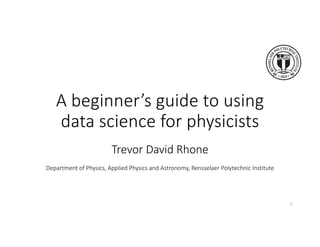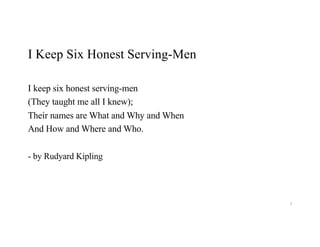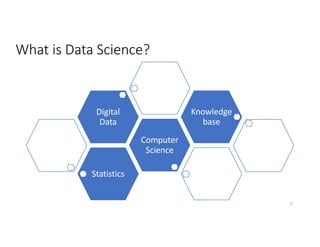Successfully reported this slideshow.

# APS GDS data science talk by Trevor Rhone

0

Share×
1 of 35
1 of 35

# APS GDS data science talk by Trevor Rhone

0

Share

A beginner’s guide to using data science for physicists.

A beginner’s guide to using data science for physicists.

## More Related Content

### Related Books

Free with a 30 day trial from Scribd

See all

### Related Audiobooks

Free with a 30 day trial from Scribd

See all

### APS GDS data science talk by Trevor Rhone

1. 1. A beginner’s guide to using data science for physicists Trevor David Rhone Department of Physics, Applied Physics and Astronomy, Rensselaer Polytechnic Institute 1
2. 2. I Keep Six Honest Serving-Men I keep six honest serving-men (They taught me all I knew); Their names are What and Why and When And How and Where and Who. - by Rudyard Kipling 2
3. 3. What is Data Science? Statistics Computer Science Digital Data Knowledge base 3
4. 4. What is Data Science? Data Science Machine Learning Statistics Visualization Databases Data mining AI 4
5. 5. Why do we care about data science? o Netflix movie recommendations o Materials Discovery • Experiments slow • Calculations expensive • No analytical solution o Uncover physical insights 5 o Targeted advertisements o Self driving cars
6. 6. o Thales of Miletus, Ancient Greece (624 BC – 546 BC) The When and Where of data science o Age of big data • Data are accessible • Data analytics tools are accessible 6 o Observational astronomy o Bioinformatics o Social media and targeted advertising
7. 7. The essential guide How to do data science? 1. Get data o Kaggle o Google dataset search 2. What are good descriptors? o Mathematical representation of the data o Domain knowledge 3. Data visualization 4. Model selection 5. Model validation 6. Model exploitation Photo: https://commons.wikimedia.org/7
8. 8. Data Science Ecosystem How to do data science? 8
9. 9. Data science ~ data visualization + machine learning y = f(x1, x2, …, xN) + 𝜺 Inputs of machine learning modelTarget property How to do data science? Goal: learn or quantify some relationship 9
10. 10. How to do data science? o What are good descriptors? o Data visualization A. Baldominos et al., Appl. Sci. 2018, 8(11), 2321 Housing Prices 10
11. 11. How to do data science? x1 x2 x x x x x x x x x x x Supervised versus Unsupervised learning 11
12. 12. How to do data science? x1 x2 x x x x x x x x x x x Supervised versus Unsupervised learning 12
13. 13. How to do data science? x1 x2 x1 x2x x x x x x x x x x x Supervised versus Unsupervised learning 13
14. 14. How to do data science? x1 x2 x1 x2x x x x x x x x x x x Supervised versus Unsupervised learning 14
15. 15. age Student? Check rating?yes yesno yes no young middle- aged senior no no yesyes How to do data science? Statistical models 15
16. 16. Machine learning models: Regression x y Goal: Build predictive model Training data How to do data science? 𝑓(𝑥) = 𝑚𝑥 + 𝑐 16
17. 17. ethen8181.github.io How to do data science? Model validation techniques
18. 18. x y Goal: Build predictive model Training data Test data How to do data science? 𝑓(𝑥) = 𝑚𝑥 + 𝑐 Machine learning models: Regression 18
19. 19. G.A. Landrum, H. Genin, Journal of Solid State Chemistry 176 (2003) 587–593 Ferromagnetism in ordered binary transition metal alloys Machine learning models: Classification How to do data science? 19
20. 20. G.A. Landrum, H. Genin, Journal of Solid State Chemistry 176 (2003) 587–593 Ferromagnetism in ordered binary transition metal alloys Machine learning models: Classification How to do data science? 20
21. 21. G.A. Landrum, H. Genin, Journal of Solid State Chemistry 176 (2003) 587–593 Ferromagnetism in ordered binary transition metal alloys Machine learning models: Classification How to do data science? 21
22. 22. Overfitting How to do data science? 22
23. 23. Regularization o y = f(x) + 𝛆 o Constraints on coefficients of a model o LASSO • Linear regression with constraints: o Neural Networks • Drop out How to do data science? 23
24. 24. Hidden layer Output layer How to do data science? Neural Networks 24
25. 25. Hidden layer Output layer Andrew Ng, cs229.stanford.edu/notes/ Neural Networks 25
26. 26. Neural Networks Architectures Perceptron Feed Forward NN Deep NN Autoencoder Recurrent NN 26
27. 27. M. Mattheakis, P. Protopapas, D. Sondak, M. Di Giovanni, E. Kaxiras, arXiv:1904.08991 Neural Networks Architectures that incorporate physical principles 27
28. 28. A2B2X6 crystal structure Transition metal trichalcogenides are magnetic 2D crystals 1. Sivadas et al., PRB 91 235425 (2015) 2. C. Gong et al., Nature 546, 265 (2017) o CrGeTe3 is a ferromagnet (FM)1,2 o CrSiTe3 is a zigzag antiferromagnet (zigzag-AFM)1 Machine Learning for Materials studies A Case Study: Magnetic 2D crystals
29. 29. X = Te X = Se X = S magneticmoment[𝜇B] Magnetic moment of A2B2X6 T.D. Rhone et al., arxiv:1806.07989
30. 30. Magnetic moment, X=Te DFT 𝝁 Predicted𝝁 Training data Test data var(# spin↑ ) var(# valence e’) m axdif(# valence e’) m ean(polariz.) chem .spaceBoB m ean(#spin↑ ) Top 6 descriptors Machine learning predictions T.D. Rhone et al., arxiv:1806.07989
31. 31. Machine learning results Magnetic moment Formation Energy
32. 32. Who can do data science? o Programmers (python) o Data wranglers o Computer scientists o Statisticians 32 o Physicists!!!
33. 33. Resources Data o Kaggle o Google’s dataset search o Citrine datasets o Materials project Self-learning o Coursera • Andrew Ng (Machine learning) o Trevor Hastie (Intro to Statistical Learning) o Citrine newsletter Workshops o IPAM @ UCLA o IACS computeFest @ Harvard Data science tools o Jupyter notebook o Scikit-learn o TensorFlow o Keras o PyTorch materials-intelligence.com 33 Resources
34. 34. Outlook o Growing interest in data science o Google and Facebook seeking collaboration with physicists o Create machine learning tools with physics principles o AI for knowledge discovery • Beyond ‘black box’ model predictions • Use AI to understand physics 34
35. 35. Data science resources https://materials-intelligence.com/ For additional resources please visit: 35